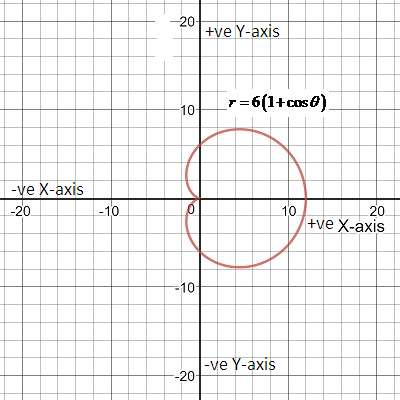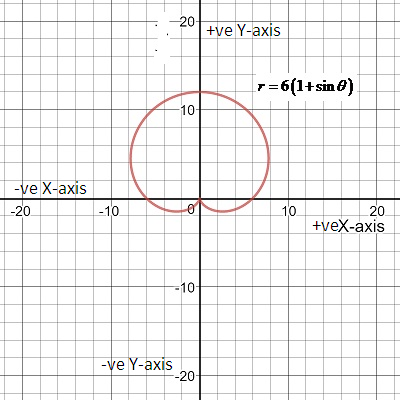Chapter 10.4, Problem 100E

Chapter
Section
Textbook Problem

Think About It Use a graphing utility to graph the polar equation r = 6 [ 1 + cos ( θ − ϕ ) ] for (a) ϕ = 0 , and (c) ϕ = π / 4 , Use the graphs to describe the effect of the angle ϕ . Write the equation as a function of sin θ for part (c).

(a)

To determine

To Graph:

The graph the polar equation r=6[1+cos(θφ)] by graphing utility when ϕ=0Explanation

Given: The given polar equation is r=6[1+cos(θφ)]

When ϕ=0 equation becomes

r=6[1+cos(θ0)]=r=6(1+Cosθ)

r=6(1+cos

(b)

To determine

To Graph:

The graph the polar equation r=6[1+cos(θφ)] by graphing utility when ϕ=π4

(c)

To determine

To Graph:

The graph the polar equation r=6[1+cos(θφ)] by graphing utility when ϕ=π2Still sussing out bartleby?

Check out a sample textbook solution.

See a sample solution

The Solution to Your Study Problems

Bartleby provides explanations to thousands of textbook problems written by our experts, many with advanced degrees!

Get Started

Draw a polygon for the distribution of scores shown in the following table. X f 6 2 5 5 4 3 3 2 2 1

Essentials of Statistics for The Behavioral Sciences (MindTap Course List)

In Exercises 39-54, simplify the expression. (Assume that x, y, r, s, and t are positive.) 41. (x2y3) (x5y3)

Applied Calculus for the Managerial, Life, and Social Sciences: A Brief Approach

CHECK POINT 2. (a) W rite as radicals. (b)Write with fractional exponents:

Mathematical Applications for the Management, Life, and Social Sciences

True or False: converges if and only if .

Study Guide for Stewart's Multivariable Calculus, 8th

Outline the major advantages and disadvantages of administering a survey by mail.

Research Methods for the Behavioral Sciences (MindTap Course List)